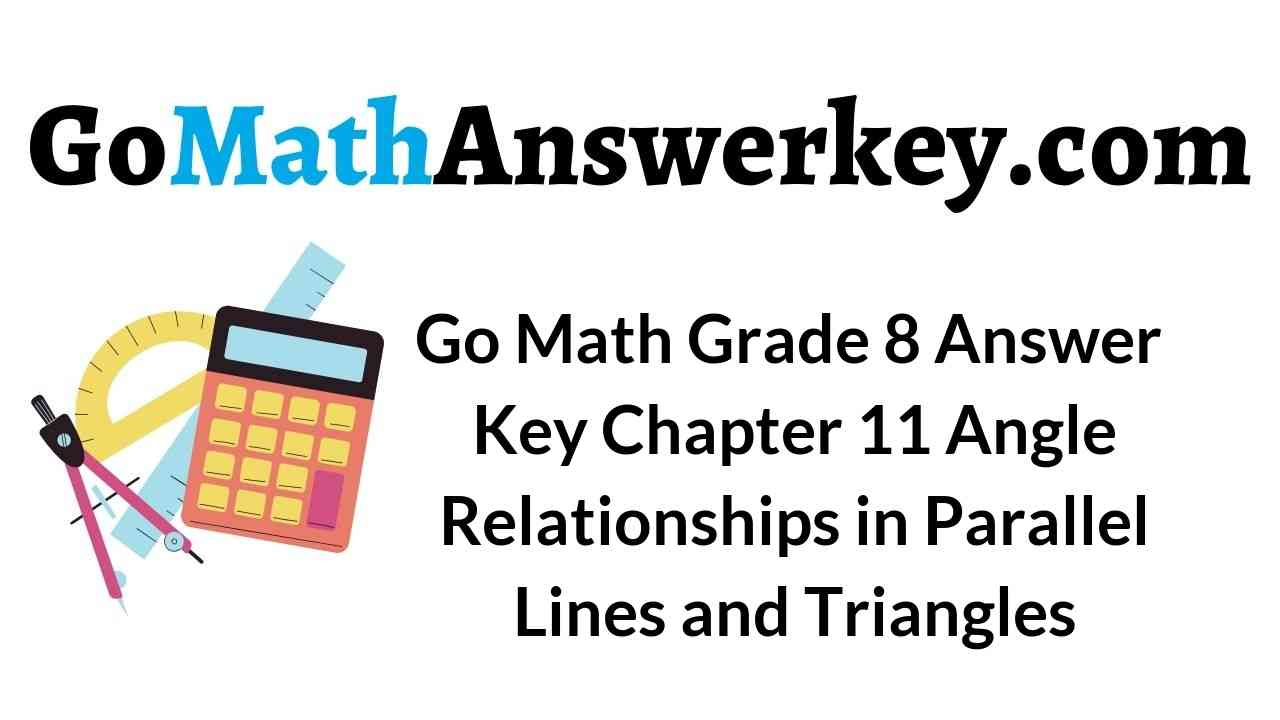# Go Math Grade 8 Answer Key Chapter 11 Angle Relationships in Parallel Lines and Triangles

## Go Math Grade 8 Chapter 11 Angle Relationships in Parallel Lines and Triangles Answer Key

Lesson 1: Parallel Lines Cut by a Transversal

Lesson 2: Angle Theorems for Triangles

Lesson 3: Angle-Angle Similarity

Model Quiz

Review

### Guided Practice – Parallel Lines Cut by a Transversal – Page No. 350

Use the figure for Exercises 1–4.Question 1.
∠UVY and ____ are a pair of corresponding angles.
∠ _________

∠ VWZ

Explanation:
∠UVY and ∠ VWZ are a pair of corresponding angles.
When two lines are crossed by Transversal the angles in matching corners are called corresponding angles.

Question 2.
∠WVY and ∠VWT are _________ angles.
____________

∠WVY and ∠VWT are alternate interior angles.
Alternate Interior Angles are a pair of angles on the inner side of each of those two lines but on opposite sides of the transversal.

Explanation:
∠WVY and ∠VWT are alternate interior angles.
Alternate Interior Angles are a pair of angles on the inner side of each of those two lines but on opposite sides of the transversal.

Parallel Lines cut by a Transversal Answer Key Question 3.
Find m∠SVW.
_________ °

80º

Explanation:
∠SVW and ∠VWT are same sider interior angles. Therefore,
m∠SVW + m∠VWT = 180º
4xº +5xº = 180º
9x = 180º
x = 180/9
x = 20
m∠SVW = 4xº = (4.20)º = 80º

Question 4.
Find m∠VWT.
_________ °

100º

Explanation:
∠SVW and ∠VWT are same sider interior angles. Therefore,
m∠SVW + m∠VWT = 180º
4xº +5xº = 180º
9x = 180º
x = 180/9
x = 20
m∠VWT = 5xº = (5.20)º = 100º

Question 5.
Vocabulary When two parallel lines are cut by a transversal, _______________ angles are supplementary.
____________

If two parallel lines are cut by a transversal, then the pairs of alternate exterior angles are congruent. If two parallel lines are cut by a transversal, then the pairs of consecutive interior angles are supplementary.

ESSENTIAL QUESTION CHECK-IN

Question 6.
What can you conclude about the interior angles formed when two parallel lines are cut by a transversal?
Type below:
____________

Alternate interior angles are congruent; same-side interior angles are supplementary.

Explanation:
When two parallel lines are cut by a transversal, the interior angles will be the angles between the two parallel lines. Alternate interior angles will be on opposite sides of the transversal; the measures of these angles are the same.
Same-side interior angles will be on the same side of the transversal; the measures of these angles will be supplementary, adding up to 180 degrees.

### 11.1 Independent Practice – Parallel Lines Cut by a Transversal – Page No. 351

Vocabulary Use the figure for Exercises 7–10.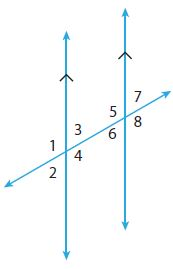Question 7.
Name all pairs of corresponding angles.
Type below:
____________

∠1 and ∠5
∠3 and ∠7
∠2 and ∠6
∠4 and ∠8

Explanation:
Corresponding angles are
∠1 and ∠5
∠3 and ∠7
∠2 and ∠6
∠4 and ∠8

Parallel Lines cut by a Transversal Worksheet Answer Key Question 8.
Name both pairs of alternate exterior angles.
Type below:
____________

∠1 and ∠8
∠2 and ∠7

Explanation:
Alternate exterior angles
∠1 and ∠8
∠2 and ∠7

Question 9.
Name the relationship between ∠ 3 and ∠6.
Type below:
____________

alternate interior angles

Explanation:
∠3 and ∠6 are alternate interior angles.
Alternate Interior Angles are a pair of angles on the inner side of each of those two lines but on opposite sides of the transversal.

Question 10.
Name the relationship between ∠4 and ∠6.
Type below:
____________

same-side interior angles

Explanation:
∠4 and ∠6 are same-side interior angles.

Find each angle measure.Question 11.
m∠AGE when m∠FHD = 30°
_________ °

m∠AGE = 30°

Explanation:
∠AGE and ∠FHD are alternate exterior angles.
Therefore, m∠AGE = m∠FHD = 30°
m∠AGE = 30°

Question 12.
m∠AGH when m∠CHF = 150°
_________ °

150°

Explanation:
∠AGH and ∠CHF are corresponding angles.
Therefore, m∠AGH = m∠CHF = 150°
m∠AGH = 150°

Question 13.
m∠CHF when m∠BGE = 110°
_________ °

110°

Explanation:
∠CHF and ∠BGE are alternate exterior angles.
Therefore, m∠CHF = m∠BGE = 110°
m∠CHF = 110°

Question 14.
m∠CHG when m∠HGA = 120°
_________ °

m∠CHG = 60º

Explanation:
∠CHF and ∠HGA are same-side interior angles.
m∠CHG + m∠HGA = 180°
m∠CHG + 120° = 180°
m∠CHG = 180 – 120 = 60
m∠CHG = 60º

Question 15.
m∠BGH
_________ °

78º

Explanation:
∠BGH and ∠GHD are same-side interior angles.
So, ∠BGH + ∠GHD = 180º
3x + (2x + 50)º = 180º
5x = 180º – 50º = 130º
x = 130/5 = 26º
∠BGH = 3xº = 3 × 26º = 78º
∠GHD = (2x + 50) += (2 × 26 + 50) = 102º

Question 16.
m∠GHD
_________ °

102º

Explanation:
∠BGH and ∠GHD are same-side interior angles.
So, ∠BGH + ∠GHD = 180º
3x + (2x + 50)º = 180º
5x = 180º – 50º = 130º
x = 130/5 = 26º
∠BGH = 3xº = 3 × 26º = 78º
∠GHD = (2x + 50) += (2 × 26 + 50) = 102º

Parallel Lines cut by a Transversal Quiz Answer Key Question 17.
The Cross Country Bike Trail follows a straight line where it crosses 350th and 360th Streets. The two streets are parallel to each other. What is the measure of the larger angle formed at the intersection of the bike trail and 360th Street? Explain.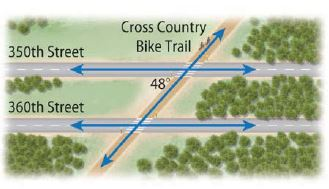_________ °

The larger angle formed at the intersection of the bike trail and 360th Street is 132º

Explanation: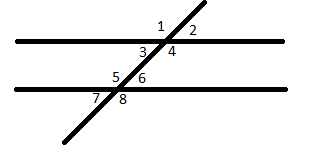The larger angle formed at the intersection of the bike trail and 360th Street is angle 5 in our schema. ∠5 and ∠3 are same-side interior angles. Therefore, m∠5 + m∠3 = 180º
m∠5 + 48º = 180º
m∠5 = 180º – 48º
m∠5 = 132º

Question 18.
Critical Thinking How many different angles would be formed by a transversal intersecting three parallel lines? How many different angle measures would there be?
_________ different angles
_________ different angle measures

12 different angles
2 different angle measures

Explanation:
There are 12 different angles formed by a transversal intersecting three parallel lines.
There are 2 different angle measures:
m∠1 = m∠4 = m∠5 = m∠8 = m∠9 = m∠12
m∠2 = m∠3 = m∠6 = m∠7 = m∠10 = m∠11

### Parallel Lines Cut by a Transversal – Page No. 352

Question 19.
Communicate Mathematical Ideas In the diagram at the right, suppose m∠6 = 125°. Explain how to find the measures of each of the other seven numbered angles.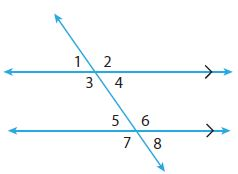Type below:
____________

m∠2 = m∠6 = 125º because ∠2 and ∠6 are corresponding angles.
m∠3 = m∠2 = 125º because ∠3 and ∠2 are vertical angles.
m∠7 = m∠3 = 125º because ∠7 and ∠3 are corresponding angles.
∠4 and ∠6 are same-side interior angles.
Therefore, m∠4 + m∠6 = 180º
m∠4 + 125º = 180º
m∠4 = 180º – 125º
m∠4 = 55º
m∠8 = m∠4 = 55º because ∠8 and ∠4 are corresponding angles.
m∠1 = m∠4 = 55º because ∠1 and ∠4 are vertical angles.
m∠5 = m∠1 = 55º because ∠5 and ∠1 are corresponding angles.

FOCUS ON HIGHER ORDER THINKING

Parallel Lines and Triangles Worksheet Answers Question 20.
Draw Conclusions In a diagram showing two parallel lines cut by a transversal, the measures of two same-side interior angles are both given as 3x°. Without writing and solving an equation, can you determine the measures of both angles? Explain. Then write and solve an equation to find the measures.

m∠1 and m∠2 are same-side interior angles is 180º
Therefore, m∠1 + m∠2 = 180º
3x + 3x = 180º
6x = 180º
x = 180/6 = 30
m∠1 = m∠2 = 3x = 3(30) = 90º

Question 21.
Make a Conjecture Draw two parallel lines and a transversal. Choose one of the eight angles that are formed. How many of the other seven angles are congruent to the angle you selected? How many of the other seven angles are supplementary to your angle? Will your answer change if you select a different angle?
Type below:
____________We have to select ∠a form of eight angles that are formed. There are two other angles that are congruent to the angle ∠a. Two other angles are ∠e and ∠g.
There are no supplementary to ∠a.
If we select a different angle then the answer will also change.

Question 22.
Critique Reasoning In the diagram at the right, ∠2, ∠3, ∠5, and∠8 are all congruent, and∠1, ∠4, ∠6, and ∠7 are all congruent. Aiden says that this is enough information to conclude that the diagram shows two parallel lines cut by a transversal. Is he correct? Justify your answer.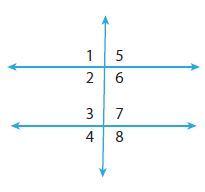____________

This is not enough information to conclude that the diagram shows two parallel lines cut by a transversal. Because ∠2 and ∠3 are same-side interior angles. But ∠5 and ∠8 are not congruent with each other. And ∠6 and ∠7 are same-side interior angles. But ∠1 and ∠4 are not congruent with each other.

### Guided Practice – Angle Theorems for Triangles – Page No. 358

Find each missing angle measure.

Question 1.m∠M = _________ °

m∠M = 71º

Explanation:
From the Triangle Sum Theorem,
m∠L + m∠N + m∠M = 180º
78º + 31º + m∠M = 180º
109º + m∠M = 180º
m∠M = 180º – 109º
m∠M = 71º

Angle Relationships Study Guide Answer Key Pdf Question 2.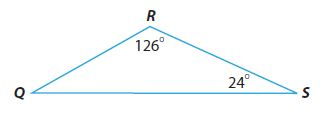m∠Q = _________ °

m∠Q = 30º

Explanation:
From the Triangle Sum Theorem,
m∠Q + m∠S + m∠R = 180º
m∠Q + 24º + 126º = 180º
m∠Q + 150º = 180º
m∠Q = 180º – 150º
m∠Q = 30º

Use the Triangle Sum Theorem to find the measure of each angle in degrees.

Question 3.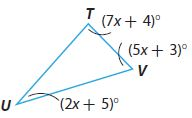m∠T = _________ °
m∠V = _________ °
m∠U = _________ °

m∠T = 88°
m∠V = 63°
m∠U = 29°

Explanation:
From the Triangle Sum Theorem,
m∠U + m∠T + m∠V = 180º
(2x + 5)º + (7x + 4)º + (5x + 3)º = 180º
2xº + 5º + 7xº + 4º + 5xº + 3º = 180º
14xº + 12º = 180º
14xº = 168º
x = 168/14 = 12
Substitute x value to find the angles
m∠U = (2x + 5)º = ((2 . 12) + 5)º = 29º
m∠U = 29º
m∠T = (7x + 4)º = ((7 . 12) + 4)º = 88º
m∠T = 88º
m∠V = (5x + 3)º = ((5 . 12) + 3)º = 63º
m∠V = 63º

Parallel Lines and Angle Relationships Worksheet Answers Question 4.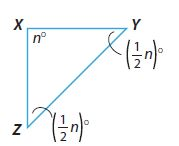m∠X = _________ °
m∠Y = _________ °
m∠Z = _________ °

m∠X = 90°
m∠Y = 45 °
m∠Z = 45°

Explanation:
From the Triangle Sum Theorem,
m∠X + m∠Y + m∠Z = 180º
nº + (1/2 . n)º + (1/2 . n)º = 180º
2nº = 180º
n = 90
Substitute n values to find the angles
m∠X = nº = 90º
m∠X = 90º
m∠Y = (1/2 . n)º = (1/2 . 90)º = 45º
m∠Y = 45º
m∠Z = (1/2 . n)º = (1/2 . 90)º = 45º
m∠Z = 45º

Use the Exterior Angle Theorem to find the measure of each angle in degrees.

Question 5.m∠C = _________ °
m∠D = _________ °

m∠C = 40°
m∠D = 76°

Explanation:
Given m∠C = 4y°, m∠D = (7y + 6)°, m∠E = 116°
By using the exterior angle theorem,
∠DEC + ∠DEF = 180°∠DEC + 116° = 180°
∠E = ∠DEC = 180° – 116° = 64°
The sum of the angles of a triangle = 180°
∠C +∠D + ∠E = 180°
4y° + (7y + 6)°+ 64° = 180°
11y° + 70° = 180°
11y° = 180° – 70° = 110°
y = 10
∠C = 4y° = 4. 10 = 40°
∠D = (7y + 6)° = ((7 . 10)  + 6)° = (70 + 6)° = 76°

Angle Relationships Worksheet Answer Key Pdf Question 6.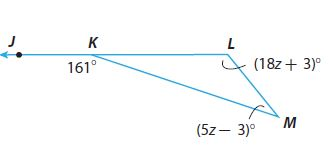m∠L = _________ °
m∠M = _________ °

m∠L = 129°
m∠M = 32°

Explanation:
Given that m∠M = (5z – 3)°, m∠L = (18z + 3)°, m∠JKM = 161°
From the Exterior Angle Theorem,
m∠M + m∠L = m∠JKM
(5z – 3)° + (18z + 3)° = 161°
5z° – 3° + 18z° + 3° = 161°
23z° = 161°
z = 161/23 = 7
Substitute z values to find the angles
m∠M = (5z – 3)° = ((5 . 7) – 3)° = 32°
m∠L = (18z + 3)° = ((18 . 7) + 3)° = 129°
From the Triangle Sum Theorem,
m∠M + m∠L + m∠LKM = 180º
32º + 129º + m∠LKM = 180º
161º + m∠LKM = 180º
m∠LKM = 19º

ESSENTIAL QUESTION CHECK-IN

Question 7.
Describe the relationships among the measures of the angles of a triangle.
Type below:
______________

The sum of all measures of the interior angles of a triangle is 180°. The measure of the exterior angle of a triangle is equal to the sum of its remote interior angles.

### 11.2 Independent Practice – Angle Theorems for Triangles – Page No. 359

Find the measure of each angle.

Question 8.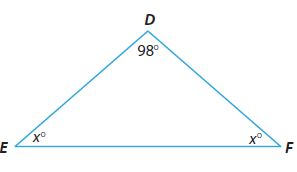m∠E = _________ °
m∠F = _________ °

m∠E = 41°
m∠F = 41°

Explanation:
m∠E = x°, m∠F = x°,  m∠D = 98°
From the Triangle Sum Theorem, sum of the angles of the traingle is 180°
m∠E + m∠D + m∠F = 180°
x + 98 + x = 180°
2x + 98 = 180°
2x = 82°
x = 41°
So, m∠E = 41°
m∠F = 41°

Angle Relationships in Triangles Answer Key Question 9.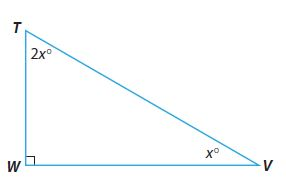m∠T = _________ °
m∠V = _________ °

m∠T = 60°
m∠V = 30°

Explanation:
m∠W = 90°, m∠T = 2x°,  m∠V = x°
From the Triangle Sum Theorem, the sum of the angles of the triangle is 180°
m∠T + m∠V + m∠W = 180°
2x + x + 90 = 180°
3x = 90°
x = 30°
So, m∠T = 2x° = 2 . 30° = 60°
m∠V = x° = 30°

Question 10.m∠G = _________ °
m∠H = _________ °
m∠J = _________ °

m∠G = 75°
m∠H = 60°
m∠J = 45°

Explanation:
m∠G = 5x°, m∠H = 4x°,  m∠J = 3x°
From the Triangle Sum Theorem, the sum of the angles of the traingle is 180°
m∠G + m∠H + m∠J = 180°
5x + 4x + 3x = 180°
12x = 90°
x = 15°
So, m∠G = 5x° = 5 . 15° = 75°
m∠H = 4x° = 4. 15° = 60°
m∠J = 3x° = 3. 15° = 45°

Question 11.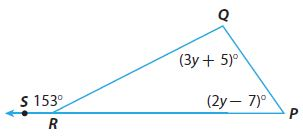m∠Q = _________ °
m∠P = _________ °
m∠QRP = _________ °

m∠Q = 98°
m∠P = 55°
m∠QRP = 27°

Explanation:
Given that m∠Q = (3y + 5)°, m∠P = (2y – 7)°, m∠QRS = 153°
From the exterior angle Theorem,
∠QRS + ∠QRP = 180°
153° + ∠QRP = 180°m∠R = m∠QRP = 180° – 153° = 27°
From the Triangle Sum Theorem, the sum of the angles of the triangle is 180°
m∠P + m∠Q + m∠R = 180°
(3y + 5)° + (2y – 7)°+ 27° = 180°
5y° + 25 = 180°
5y° = 155°
y = 31°
m∠Q = (3y + 5)° = ((3 . 31°) + 5)° = 98°
m∠P = (2y – 7)° = ((2. 31° – 7)° = 55°
m∠QRP = 27°

Angle Relationships Math Lib Answer Key Question 12.m∠ACB = _________ °
m∠DCE = _________ °
m∠BCD = _________ °

m∠ACB = 44°
m∠DCE = 35°
m∠BCD = 101°

Explanation:
In traingle ABC, m∠A = 78°, m∠B = 58°, m∠ACB = ?°
From the Triangle Sum Theorem, the sum of the angles of the triangle is 180°
m∠A + m∠B + m∠ACB = 180°
78° + 58° + m∠ACB = 180°
m∠ACB = 180° – 136°
m∠ACB = 44°
In traingle CDE, m∠D = 85°, m∠E = 60°, m∠CDE = ?°
From the Triangle Sum Theorem, the sum of the angles of the triangle is 180°
m∠D + m∠E + m∠CDE = 180°
85° + 60° + m∠CDE = 180°
m∠CDE = 180° – 145°
m∠CDE = 35°
From the Exterior Angle Theorem,
m∠ACB + m∠CDE + m∠BCD = 180°
44° + 35° + m∠BCD = 180°
m∠BCD = 180° – 79°
m∠BCD = 101°

Question 13.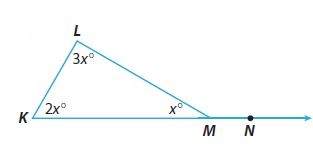m∠K = _________ °
m∠L = _________ °
m∠KML = _________ °
m∠LMN = _________ °

Explanation:
m∠K = 2x°, m∠L = 3x°, m∠KML = x°
So, From the Triangle Sum Theorem, the sum of the angles of the triangle is 180°.
m∠K + m∠L + m∠KML = 180°
2x° + 3x° + x° = 180°
6x° = 180°
x= 30°
∠KML = x = 30°
∠L = 3x = 3 . 30° = 90°
∠K = 2x = 2 . 30° = 60°
From the Exterior Angle Theorem,
∠KML + ∠LMN = 180°
∠LMN = 180° – 30° = 150°

Question 14.
Multistep The second angle in a triangle is five times as large as the first. The third angle is two-thirds as large as the first. Find the angle measures.
The measure of the first angle: _________ °
The measure of the second angle: _________ °
The measure of the third angle: _________ °

The measure of the first angle: 27°
The measure of the second angle: 135°
The measure of the third angle: 18°

Explanation:
Let us name the angles of a triangle as ∠1, ∠2, ∠3.
Consider ∠1 as x.
∠2 is 5 times as large as the first.
∠2 = 5x
Also, ∠3 = 2/3 . x
So, From the Triangle Sum Theorem, the sum of the angles of the triangle is 180°.
x+ 5x + (2/3 . x) = 180°
20x = 540°
x = 27°
So, ∠1 = x = 27°
∠2 = 5x = 5 . 27° = 135°
∠3 = 2/3 . x = 2/3 . 27° = 18°
The measure of the first angle: 27°
The measure of the second angle: 135°
The measure of the third angle: 18°

### Angle Theorems for Triangles – Page No. 360

Question 15.
Analyze Relationships Can a triangle have two obtuse angles? Explain.
___________

No; a triangle can NOT have two obtuse angles

Explanation:
The measure of an obtuse angle is greater than 90°. Two obtuse angles and the third angle would have a sum greater than 180°

FOCUS ON HIGHER ORDER THINKING

Question 16.
Critical Thinking Explain how you can use the Triangle Sum Theorem to find the measures of the angles of an equilateral triangle.
Type below:
___________

All angles have the same measure in an equilateral triangle

Explanation:
Using the Triangle Sum Theorem,
∠x + ∠x + ∠x = 180°
3∠x = 180°
∠x = 60°
All angles have the same measure in an equilateral triangle

Angle Relationships 8th Grade Math Question 17.
a. Draw Conclusions Find the sum of the measures of the angles in quadrilateral ABCD. (Hint: Draw diagonal $$\overline { AC }$$. How can you use the figures you have formed to find the sum?)Sum: _________ °

Sum: 360°

Question 17.
b. Make a Conjecture Write a “Quadrilateral Sum Theorem.” Explain why you think it is true.
Type below:
___________

The sum of the angle measures of a quadrilateral is 360°
Any quadrilateral can be divided into two triangles (180 + 180 = 360)

Angle in Triangles Math Lib Answer Key Question 18.
Communicate Mathematical Ideas Describe two ways that an exterior angle of a triangle is related to one or more of the interior angles.
Type below:
___________

An exterior angle and it’s an adjacent interior angle equal 180°
An exterior angle equals the sum of the two remote interior angles.

### Guided Practice – Angle-Angle Similarity – Page No. 366

Question 1.
Explain whether the triangles are similar. Label the angle measures in the figure.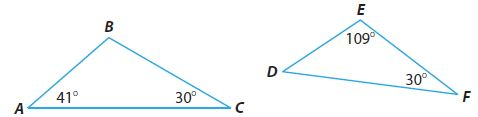Type below:
___________
△ABC has angle measures _______and △DEF has angle measures______. Because _______in one triangle are congruent to ______in the other triangle, the triangles are_____.

△ABC has angle measures 40°, 30°, and 109° and △DEF has angle measures 41°, 109°, and 30°. Because 2∠s in one triangle are congruent to in the other triangle, the triangles similar.

Angle-Angle Similarity Worksheet Answers Question 2.
A flagpole casts a shadow 23.5 feet long. At the same time of day, Mrs. Gilbert, who is 5.5 feet tall, casts a shadow that is 7.5 feet long. How tall in feet is the flagpole? Round your answer to the nearest tenth.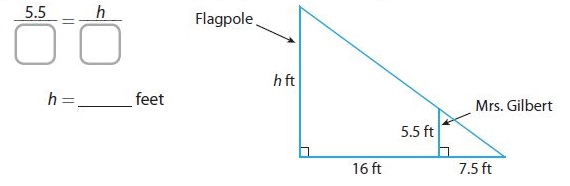_________ ft

17.2 ft

Explanation:
In similar triangles, corresponding side lengths are proportional.
5.5/7.5 = h/23.5
h (7.5) = 129.25
h = 129.25/7.5
h = 17.23
Rounding to the nearest tenth
h = 17.2 feet

Question 3.
Two transversals intersect two parallel lines as shown. Explain whether △ABC and △DEC are similar.∠BAC and∠EDC are ___________ since they are ___________.
∠ABC and∠DEC are ___________ since they are ___________.
By ________, △ABC and△DEC are ___________.
Type below:
___________

∠BAC and∠EDC are congruent since they are alt. interior ∠s
∠ABC and∠DEC are congruent since they are alt. interior ∠s.
By AA similarity, △ABC and△DEC are similar.

ESSENTIAL QUESTION CHECK-IN

Question 4.
How can you determine when two triangles are similar?
Type below:
___________

If 2 angles of one triangle are congruent to 2 angles of another triangle, the triangles are similar by the Angle-Angle Similarity Postulate

### 11.3 Independent Practice – Angle-Angle Similarity – Page No. 367

Use the diagrams for Exercises 5–7.Question 5.
Find the missing angle measures in the triangles.
Type below:
___________

m∠B = 42°
m∠F = 69°
m∠H = 64°
m∠K = 53°

Explanation:
Using the Triangle Sum Theorem,
m∠A + m∠B + m∠C = 180°
85° + m∠B + 53° = 180°
138° + m∠B = 180°
m∠B = 180° – 138°
m∠B = 42°
Using the Triangle Sum Theorem,
m∠D + m∠E + m∠F = 180°
We substitute the given angle measures and we solve for m∠F
64° + 47° + m∠F = 180°
111° + m∠F = 180°
m∠F = 180° – 111°
m∠F = 69°
Using the Triangle Sum Theorem,
m∠G + m∠H + m∠J = 180°
We substitute the given angle measures and we solve for m∠H
47° + m∠H + 69° = 180°
116° + m∠H = 180°
m∠H = 180° – 116°
m∠H = 64°
Using the Triangle Sum Theorem,
m∠J + m∠K + m∠L = 180°
We substitute the given angle measures and we solve for m∠K
85° + m∠K + 42° = 180°
127° + m∠K = 180°
m∠K = 180° – 127°
m∠K = 53°

11.3 Corresponding Parts of Similar Figures Answer Key Pdf Question 6.
Which triangles are similar?
Type below:
___________

△ABC and △JKL are similar because their corresponding angles are congruent. Also, △DEF and △GHJ are similar because their corresponding is congruent.

Question 7.
Analyze Relationships Determine which angles are congruent to the angles in △ABC.
∠A ≅ ∠ ________
∠B ≅ ∠ ________
∠C ≅ ∠ ________

△JKL ≅ △ABC

Explanation:
△JKL has angle measures that are the same as those is △ABC
∠A ≅ ∠ J
∠B ≅ ∠ L
∠C ≅ ∠ K
Therefore, they are congruent.

Question 8.
Multistep A tree casts a shadow that is 20 feet long. Frank is 6 feet tall,and while standing next to the tree he casts a shadow that is 4 feet long.a. How tall is the tree?
h = ________ ft

h = 30 ft

Explanation:
In similar triangles, corresponding side lengths are proportional.
20/4 = h/6
5 = h/6
h = 30
The tree is 30 feet tall.

Question 8.
b. How much taller is the tree than Frank?
________ ft

24 ft

Explanation:
30 – 6 = 24
The tree is 24 feet taller than Frank.

Question 9.
Represent Real-World Problems Sheila is climbing on a ladder that is attached against the side of a jungle gym wall. She is 5 feet off the ground and 3 feet from the base of the ladder, which is 15 feet from the wall. Draw a diagram to help you solve the problem. How high up the wall is the top of the ladder?
________ ft

25 ft

Explanation: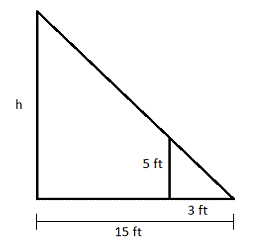3/15 = 5/h
15 ×3 = 3h
75 = 3h
h = 75/3 = 25

11.3 Practice Angle Relationships and Parallel Lines Answers Question 10.
Justify Reasoning Are two equilateral triangles always similar? Explain.
______________

yes; two equilateral triangles are always similar.
Each angle of an equilateral triangle is 60°. Since both triangles are equilateral then they are similar.

### Angle-Angle Similarity – Page No. 368

Question 11.
Critique Reasoning Ryan calculated the missing measure in the diagram shown. What was his mistake?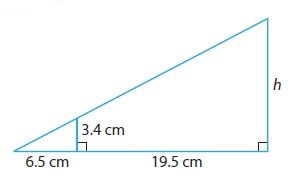$$\frac{3.4}{6.5}=\frac{h}{19.5}$$
19.5 × $$\frac{3.4}{6.5}=\frac{h}{19.5}$$ × 19.5
$$\frac{66.3}{6.5}$$ = h
10.2cm = h
Type below:
___________

In the first line, Ryan did not take the sum of 6.5 and 19.5 to get the denominator on the right.
The denominator on the right should be 26 instead of 19.5
The correct value for h
3.4/6.5 = h/26
h = (3.4/6.5) × 26
h = 13.6cm

FOCUS ON HIGHER ORDER THINKING

Question 12.
Communicate Mathematical Ideas For a pair of triangular earrings, how can you tell if they are similar? How can you tell if they are congruent?
Type below:
___________

The earrings are similar if two angle measures of one are equal to two angle measures of the other.
The earrings are congruent if they are similar and if the side lengths of one are equal to the side lengths of the other.

Question 13.
Critical Thinking When does it make sense to use similar triangles to measure the height and length of objects in real life?
Type below:
___________

If the item is too tall or the distance is too long to measure directly, similar triangles can help with measuring.

Question 14.
Justify Reasoning Two right triangles on a coordinate plane are similar but not congruent. Each of the legs of both triangles are extended by 1 unit, creating two new right triangles. Are the resulting triangles similar? Explain using an example.
___________

Two triangles are similar if their corresponding angles are congruent and the lengths of their corresponding sides are proportional. If each of the legs of both triangles is extended by 1 unit, the ratio between proportional sides does not change. Therefore, the resulting triangles are similar.

### Ready to Go On? – Model Quiz – Page No. 369

11.1 Parallel Lines Cut by a Transversal

In the figure, line p || line q. Find the measure of each angle if m∠8 = 115°.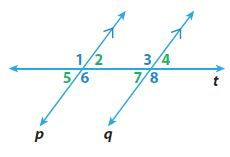Question 1.
m∠7 = _________ °

m∠7 = 65°

Explanation:
According to the exterior angle theorem,
m∠7 + m∠8 = 180°
m∠7 + 115° = 180°
m∠7 = 180° – 115°
m∠7 = 65°

How do Parallel Lines cut by a Transversal Question 2.
m∠6 = _________ °

m∠6 = 115°

Explanation:
From the given figure, Line P is parallel to line Q. So, the angles given in line P is equal to the angles in line Q. They are corresponding angles.
So, m∠8 is parallel is m∠6 or m∠8 = m∠6 = 115°

Question 3.
m∠1 = _________ °

m∠1 = 115°

Explanation:
∠1 and ∠6 are alternative exterior angles.
So, m∠1 = m∠6 = 115°

11.2 Angle Theorems for Triangles

Find the measure of each angle.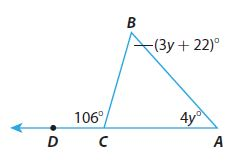Question 4.
m∠A = _________ °

m∠A = 48°

Explanation:
m∠A + m∠B + m∠C = 180°
4y° + (3y + 22)° + 74° = 180°
7y = 180 – 96 = 84
y = 12°
m∠A = 4y° = 4 (12°) = 48°
m∠B = (3y + 22)° = (3(12°) + 22)° = 58°

Question 5.
m∠B = _________ °

m∠B = 58°

Explanation:
m∠A + m∠B + m∠C = 180°
4y° + (3y + 22)° + 74° = 180°
7y = 180 – 96 = 84
y = 12°
m∠A = 4y° = 4 (12°) = 48°
m∠B = (3y + 22)° = (3(12°) + 22)° = 58°

Transversal Angles Relationships Question 6.
m∠BCA = _________ °

m∠BCA = 74°

Explanation:
m∠BCD + m∠BCA = 180°
106° + m∠BCA = 180°
m∠BCA = 180° – 106°
m∠BCA = 74°
So, m∠BCA = 74°

11.3 Angle-Angle Similarity

Triangle FEG is similar to triangle IHJ. Find the missing values.Question 7.
x = _________

x = 16

Explanation:
In similar triangles, corresponding side lengths are proportional.
HJ/EG = IJ/FG
(x + 12)/42 = 40/60
(x + 12)/42 = 4/6
6x = 96
x = 16

Question 8.
y = _________

y = 9

Explanation:
In similar triangles, corresponding side lengths are congruent.
m∠HJI = m∠EGF
(5y + 7)° = 52°
5y° + 7° = 52°
5y° = 45°
y = 9

Question 9.
m∠H = _________°

m∠H = 92°

Explanation:
Using the Triangle Sum Theorem,
m∠E + m∠F + m∠G = 180°
We substitute the given angle measures and we solve for m∠E
m∠E + 36° + 52° = 180°
m∠E + 88° = 180°
m∠E = 92°
In similar angles, corresponding side lengths are congruent
m∠H = m∠E
m∠H = 92°

ESSENTIAL QUESTION

Question 10.
How can you use similar triangles to solve real-world problems?
Type below:
____________

we know that if two triangles are similar, then their corresponding angles are congruent and the lengths of their corresponding sides are proportional. We can use this to determine values that we cannot measure directly. For example, we can calculate the length of the tree if we measure its shadow and our shadow on a sunny day.

### Selected Response – Mixed Review – Page No. 370

Use the figure for Exercises 1 and 2.Question 1.
Which angle pair is a pair of alternate exterior angles?
Options:
A. ∠5 and ∠6
B. ∠6 and∠7
C. ∠5 and ∠4
D. ∠5 and ∠2

C. ∠5 and ∠4

Explanation:
∠5 and ∠4 are alternate exterior angles

Question 2.
Which of the following angles is not congruent to ∠3?
Options:
A. ∠1
B. ∠2
C. ∠6
D. ∠8

B. ∠2

Explanation:
∠2 and ∠3 are same-side interior angles. They are not congruent instead their sum is equal to 180°

Angle Sums and Exterior Angles of Triangles Independent Practice Worksheet Answers Question 3.
The measures, in degrees, of the three angles of a triangle are given by 2x + 1, 3x – 3, and 9x. What is the measure of the smallest angle?
Options:
A. 13°
B. 27°
C. 36°
D. 117°

B. 27°

Explanation:
From the Triangle Sum Theorem, the sum of the angles of the triangle is 180°
m∠1 + m∠2 + m∠3 = 180°
(2x + 1)° + (3x – 3)° + (9x)° = 180°
2x° + 1° + 3x° – 3° + 9x° = 180°
14x° – 2° = 180°
14x° = 178°
x = 13
Substitute the value of x to find the m∠1, m∠2, and m∠3
m∠1 = (2x + 1)° = (2(13) + 1)° = 27°
m∠2 = (3x – 3)° = (3(13) – 3)° = 36°
m∠3 = (9x)° = (9(13))° = 117°
The smallest angle is 27°

Question 4.
Which is a possible measure of ∠DCA in the triangle below?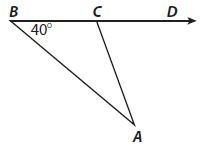Options:
A. 36°
B. 38°
C. 40°
D 70°

D 70°

Explanation:
Using the Exterior Angle Theorem
m∠A + m∠B = m∠DCA
m∠A + 40° = m∠DCA
m∠DCA will be greater than 40°. The only suitable option is D, 70°.

Question 5.
Kaylee wrote in her dinosaur report that the Jurassic period was 1.75 × 108 years ago. What is this number written in standard form?
Options:
A. 1,750,000
B. 17,500,000
C. 175,000,000
D. 17,500,000,000

C. 175,000,000

Explanation:
1.75 × 108 standard form
Move the decimal point to 8 right places.
175,000,000

Question 6.
Given that y is proportional to x, what linear equation can you write if y is 16 when x is 20?
Options:
A. y = 20x
B. y = $$\frac{5}{4}$$ x
C. y = $$\frac{4}{5}$$x
D. y = 0.6x

C. y = $$\frac{4}{5}$$x

Explanation:
Y=4/5x
16=4/5(20)
4/5×20/1=80/5
80/5=16

Angles and Parallel Lines Answer Key Question 7.
Two transversals intersect two parallel lines as shown.a. What is the value of x?
x = ________

x = 4

Explanation:
mJKL = mLNM
6x + 1 = 25
6x = 24
x = 4

Question 7.
b. What is the measure of ∠LMN?
_________°

23°

Explanation:
m∠LMN = 3x + 11 = 3(4) + 11 = 12 + 11 = 23

Question 7.
c. What is the measure of ∠KLM?
∠KLM = _________°

∠KLM = 48°

Explanation:
∠KLM exterior angle of the triangle LMN
m∠KLM = m∠LNM + m∠LMN
= 25 + 23 = 48

Question 7.
d. Which two triangles are similar? How do you know?
Type below:
_____________